# Chromatic number of the plane

The unit distance graph on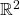has edges between those pairs of points at Euclidean distance.  The chromatic number of this graph lies between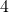(by exhibiting a small subgraph on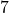vertices with chromatic number) and(by an explicit colouring based on a hexagonal tiling of the plane).  Aubrey de Grey has just posted a construction of a unit distance graph with chromatic number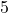, raising the lower bound on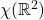byThis MathOverflow post is a good jumping off point into the discussion online.

I was explaining this problem to a colleague and they asked whether this graph was connected (it is) and whether that was still true if we restricted to rational coordinates.  It turns out this was addressed by Kiran B.Chilakamarri in 1988, and the answer is the rational unit distance graph is connected from dimensiononwards.

To see that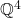is not connected, consider a general unit vector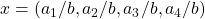where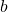is coprime to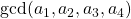.  Then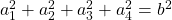.

Claim.is divisible by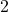at most once.

Proof. Squares mod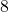are either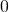,or.  Ifis divisible bythen one of the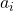is odd, hence squares tomod.  But then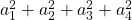cannot be divisible by, which is a contradiction.

So the entries of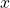in their reduced form do not contain any‘s in their denominator, and so the same must hold for all sums of unit vectors.  Hence we can’t express, say,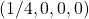as a sum of unit vectors, andis not connected to.

Connectedness in dimension(hence also later) uses Lagrange’s theorem on the sums of four squares.  We’ll show that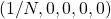can be expressed as a sum ofunit vectors.  By Lagrange’s theorem, write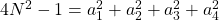.  Then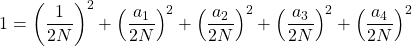hence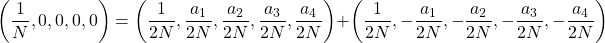is a sum ofunit vectors.